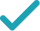Type a math problemSolve for xSteps for Solving Linear Equation
Variable cannot be equal to since division by zero is not defined. Multiply both sides of the equation by .
Subtract from both sides.
Combine and to get .
Add to both sides. Anything plus zero gives itself.
Divide both sides by .
Expand by multiplying both numerator and the denominator by .
Reduce the fraction to lowest terms by extracting and canceling out .
GraphGraph Both Sides in 2D
Graph in 2DGiving is as easy as 1, 2, 3
Get 1,000 points to donate to a school of your choice when you join Give With Bing
x-120500=0.02x
Variable x cannot be equal to 0 since division by zero is not defined. Multiply both sides of the equation by x.
x-120500-0.02x=0
Subtract 0.02x from both sides.
0.98x-120500=0
Combine x and -0.02x to get 0.98x.
0.98x=120500
Add 120500 to both sides. Anything plus zero gives itself.
x=\frac{120500}{0.98}
Divide both sides by 0.98.
x=\frac{12050000}{98}
Expand \frac{120500}{0.98}\approx 122959.183673469 by multiplying both numerator and the denominator by 100.
x=\frac{6025000}{49}
Reduce the fraction \frac{12050000}{98}\approx 122959.183673469 to lowest terms by extracting and canceling out 2.